# Limesurvey: Randomly show x out of y question groups

### Proof of concept: Show x out of y question groups in random order

Limesurvey has the possibility to show x out of y groups in a random order. We created a proof of concept to show this
https://survey.toolsforresearch.com/index.php/345678?newtest=Y&lang=en

The idea for this example survey was borrowed from Tony Partner:
https://www.limesurvey.org/forum/can-i-do-this-with-limesurvey/107889-randomly-select-question-groups#145950

### The slider to choose the number of groups (variable x)

Create a 'Multiple numerical input' question with Qcode 'gcount':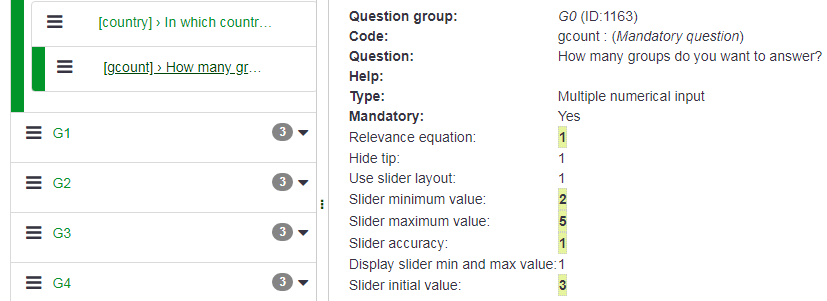The maximum of the slider should match the number of random groups within the randomization group: the 'y' variable.

Add a subquestion '1'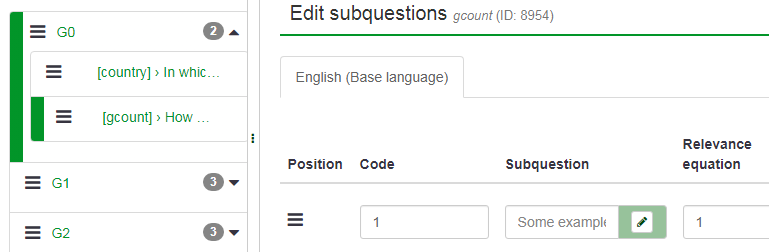The variable 'x' will now be stored in 'gcount_1'.

### Random groups

Create the random groups: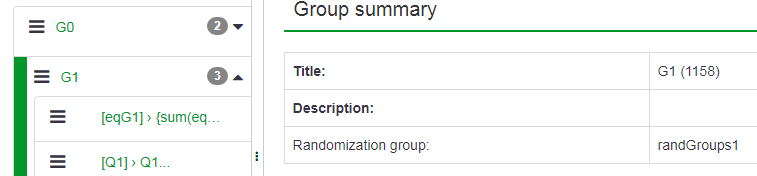Note the 'randGroups1' in Randomization group.

### Equation in each random group

Every random group should start with a hidden question of the equation type. Example for G1: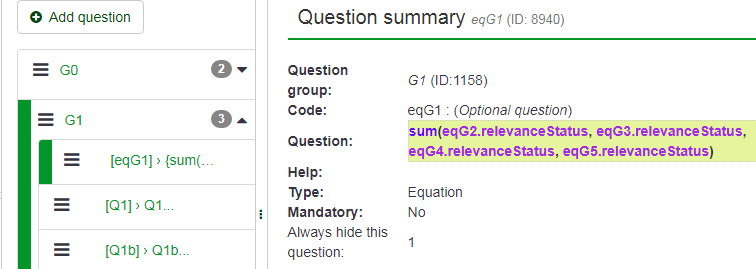Example for G2: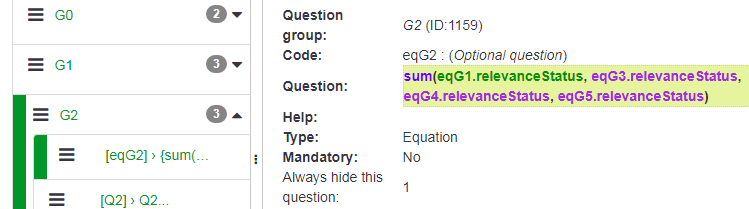As a convention we use eq+group name as Qcode for the equation in each random group: eqG1, eqG2 or eqOtherQcode

The value of the equation is the sum of the RelevanceStatuses for all the other groups in the same Randomization group.
It is stored in the Question box of the equation within curly brackets. For G1:

`{sum(eqG2.relevanceStatus, eqG3.relevanceStatus, eqG4.relevanceStatus, eqG5.relevanceStatus)}`

For G2:

`{sum(eqG1.relevanceStatus, eqG3.relevanceStatus, eqG4.relevanceStatus, eqG5.relevanceStatus)}`

Note that this can be changed without deactivating the survey. Best practice would be to use a script to enter the equation in stead of manually entering it.

### Questions within a random question group

Example of a question within G1Example of a question within G2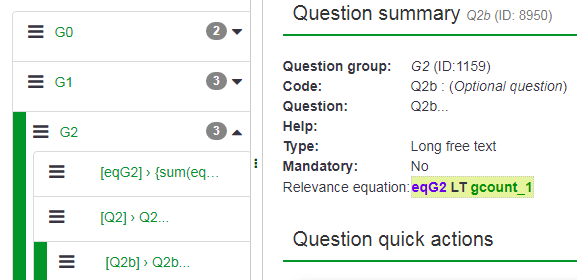The trick is that every (visible) question within each random group must have a Relevance equation that checks if the equation of the current random group is less than gcount_1 (the 'x' variable). The relevance equation is entered in the General options of each question: For the questions within the G2 group: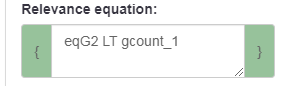Note that the Relevance equation can be changed without deactivating the survey.